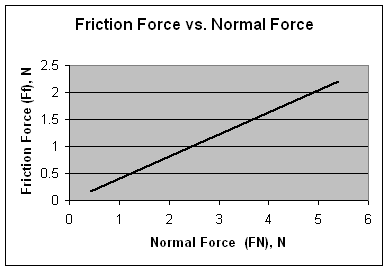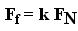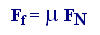#### Analyzing Friction and calculating the coefficient of friction

Equipment used:

• 1 block of wood (50 g)
• 3 standard laboratory masses (300g, 400g, 500g)
• Spring scale (preferably try to use a spring loaded scale that is susceptible to smaller weights)

Method:

1. Attach the spring scale to the block of wood loaded with a standard laboratory mass.
2. Pull the block of wood with the spring scale at a constant speed over a fixed distance of 1 meter
3. Practice step 2 a few times until you obtain a fairly constant speed before recording your answer.  This will minimize error.
4. Record the force from the spring scale

Data Collection:

 Total Mass (wood block + laboratory masses) kgm Force of gravity (wood block + laboratory masses) N= the normal force FN Force of Friction (from spring scale) NFf 350 g = 0.350 kg 0.350 kg  x 9.8 N/kg = 3.43 N 1.4 N 450 g = 0.450 kg 0.450 kg x 9.8 N/kg = 4.41 N 1.8 N 550 g = 0.550 kg 0.550 kg x 9.8 N/kg = 5.39 N 2.2 N

Analysis:

Graph the above data.

Plot the normal force FN on the x-axis and the friction Ff on the y-axis.Note: This is a straight line of the form y = kx (where k is the slope of the line).

Substitute  the symbols corresponding to our data analysis we can derive an equation relating Force of Friction to Normal Force

Therefore we can say:The constant k is obtained by calculating the slope of the line and has a value of 0.4 .

This value is known as the coefficient of friction and it is usually called  µ  (the lower case of the letter  ``m``  from the Greek alphabet,  pronounced "mu").

Conclusion:

The relationship between Force of Friction and  Normal Force can be expressed with the linear equation:This equation tells us that the higher the normal force, the higher the friction.  In other words, the higher the mass of an object the higher the friction.

It also tells us that the type of surface, according to their coefficients of friction, will have an effect of the static friction of an object moving along it.

Extention:

Research why different rubber tires have different coefficient of friction and why they are suitable for different terrains and driving conditions.